# High School Chemistry : Help with Reaction Quotient

## Example Questions

### Example Question #11 : Chemical Reactions

Consider the following balanced reaction: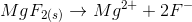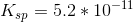The solution has the following concentrations: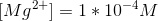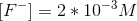Based on these concentrations, what will happen to the solution?

Possible Answers:

More information is needed in order to answer the question

It will shift to the left

The system is at equilibrium

It will shift to the right

Correct answer:

It will shift to the left

Explanation:

By placing these initial concentrations in the equilibrium expression, we can compare the reaction quotient,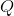, to the equilibrium constant,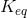The reaction quotient for this reaction is: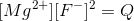Note that the reactant is a pure solid, and will not be included in this expression. By setting this equal to the equilibrium constant, we can see whetheroris larger in value.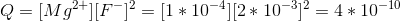We can predict how a solution will change based on the value of. Whenis less than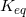, the reaction will shift to the right. Ifis greater than, the reaction will shift to the left. Ifis equal to, the solution is at equilibrium.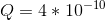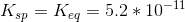Since the value foris greater than the equilibrium constant in this instance, the reaction will shift to the left.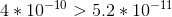### Example Question #1 : Help With Reaction Quotient

Consider the following reaction.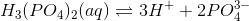What is the reaction quotient if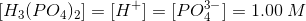?

Possible Answers: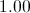There is not enough information to answer this question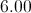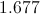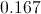Correct answer:Explanation: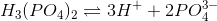The reaction quotient, or Q, of the above reaction is equal to the products over the reactants. Q is calculated in the same manner as Keq, but does not require that the reaction be at equilibrium.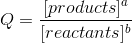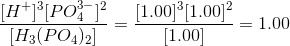### All High School Chemistry Resources